# Fatou extension

(diff) ← Older revision | Latest revision (diff) | Newer revision → (diff)

of a commutative ringA commutative ringcontainingsuch that each formal power series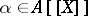which is-rational is in fact-rational. Recall that a formal power seriesis-rational,a commutative ring, if there exist two polynomials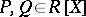such that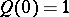and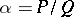, that is,is equal to the formal expansion of. For instance, ifis a field extension (cf. also Extension of a field), thenis a Fatou extension of.

Fatou extensions are well characterized in the integral case. Thus, from now on,is supposed to be an integral domain with quotient field. The example above shows that an integral domaincontainingis a Fatou extension ofif and only if the ringis a Fatou extension of. If the integral domainis Noetherian (cf. Noetherian ring), then its quotient fieldis a Fatou extension of, and, hence, every integral domain containingis a Fatou extension of. Many rings are Noetherian: for instance, every finitely generated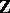-algebra is Noetherian.

For a rational function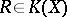, there are several representations of the form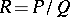with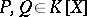. Such a representation is said to be:

a) unitary if the non-zero coefficient ofcorresponding to the lowest degree is;

b) irreducible ifandare relatively prime in(cf. also Mutually-prime numbers);

c) with coefficients inif. Let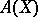denote the set of rational functions with a unitary representation with coefficients in, and let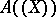denote the set of Laurent power series, that is,(these notations extend the classical notationsand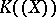).

To say that the integral domainis a Fatou extension ofis nothing else than to write:in other words, each rational function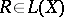, wheredenotes the quotient field of, which has a unitary representation with coefficients inand a Laurent expansion atwith coefficients in, has a unitary representation with coefficients in.

A rational functionhas a unique unitary and irreducible representation. With respect to this representation, there are two main results:

1) The ringis the set of elements of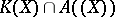which admit a unitary and irreducible representation whose coefficients are integral over.

2) For every element of, the coefficients of the unitary and irreducible representation are almost integral over. Recall that an elementofis almost integral overif there exists a non-zero elementofsuch that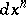belongs tofor each positive integer. Each element ofwhich is integral overis almost integral over.

An integral domaincontainingis a Fatou extension ofif and only if each element ofwhich is both integral overand almost integral overis integral over[a1]. The Noetherian case considered above follows from the fact that ifis Noetherian, then each element ofwhich is almost integral overis integral over.

The definition of Fatou extension may be easily extended to semi-ring extensions. Then,is a Fatou extension of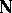, whileis not a Fatou extension of, nor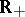of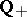[a2].

Moreover, the notion may be considered for formal power series in non-commuting variables, which have applications in system and control theory [a3]. It turns out that the previous characterization in the integral case still holds.

How to Cite This Entry:
Fatou extension. Encyclopedia of Mathematics. URL: http://encyclopediaofmath.org/index.php?title=Fatou_extension&oldid=18283
This article was adapted from an original article by Jean-Luc Chabert (originator), which appeared in Encyclopedia of Mathematics - ISBN 1402006098. See original article